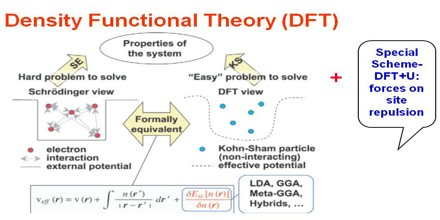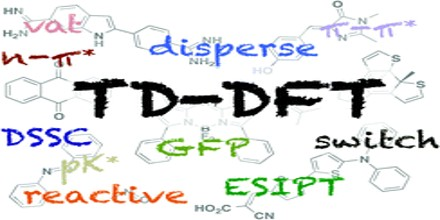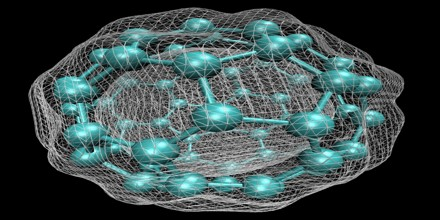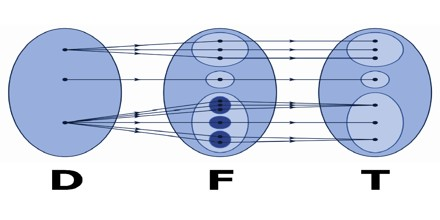DFT: Density Functional Theory

The quantum mechanical wave function contains, in principle, all the information about a given system. For the case of a simple 2-D square potential or even a hydrogen atom we can solve the Schrödinger equation exactly in order to get the wave function of the system. We can then determine the allowed energy states of the syatem. Unfortunately it is impossible to solve the Schrödinger equation for a N-body system. Evidently, we must involve some approximations to render the problem soluble albeit tricky. Here we have our simplest definition of DFT: A method of obtaining an approximate solution to the Shrodinger equation of a many-body system.DFT has proved to be highly successful in describing structural and electronic properties in a vast class of materials, ranging from atoms and molecules to simple crystals to complex extended systems (including glasses and liquids). Furthermore DFT is computationally simple. For these reasons DFT has become a common tool in first-principles calculations aimed at describing – or even predicting – properties of molecular and condensed matter systems.

DFT computational codes are used in practise to investigate the structural, magnatic and electronic properties of molecules, materials and defects.

Methods of DFT

A DFT calculation adds an additional step to each major phase of a Hartree-Fock calculation. This step is a numerical integration of the functional or various derivatives of the functional. Thus in addition to the sources of numerical error in Hartree-Fock calculations (integral accuracy, SCF convergence, CPHF convergence), the accuracy of DFT calculations also depends on the number of points used in the numerical integration.The UltraFine integration grid (corresponding to Integral=UltraFine) is the default in Gaussian 16. This grid greatly enhances calculation accuracy at reasonable additional cost. We do not recommend using any smaller grid in production DFT calculations. Note also that it is important to use the same grid for all calculations where you intend to compare energies (e.g., computing energy differences, heats of formation, and so on).

Larger grids are available when needed (e.g. tight geometry optimizations of certain kinds of systems). An alternate grid may be selected with the Integral=Grid option in the route section.

The first H–K theorem demonstrates that the ground state properties of a many-electron system are uniquely determined by an electron density that depends on only 3 spatial coordinates. It lays the groundwork for reducing the many-body problem of N electrons with 3N spatial coordinates to 3 spatial coordinates, through the use of functional of the electron density. This theorem can be extended to the time-dependent domain to develop time-dependent density functional theory (TDDFT), which can be used to describe excited states.

The second H–K theorem defines energy functional for the system and proves that the correct ground state electron density minimizes this energy functional.A major challenge in the application of block copolymer directed self-assembly (DSA) to advanced lithography is the exploration of large design spaces, including the selection of confinement shape and size, surface chemistry to affect wetting conditions, copolymer chain length and block fraction. To sweep such large spaces, a computational model is ideally both fast and accurate. In this study, we investigate various incarnations of the density functional theory (DFT) approach and evaluate their suitability to DSA applications. We introduce a new optimization scheme to capitalize on the speed advantages of DFT, while minimizing loss of accuracy relative to the benchmark of self-consistent field theory (SCFT). Although current DFT models afford a 100-fold reduction in computational complexity over SCFT, even the best optimized models fail to match SCFT density profiles and make extremely poor predictions of commensurability windows and defect energetics. These limitations suggest that SCFT will remain the gold standard for DSA simulations in the near future.Successes and Failures of DFT

DFT, even in the simplest LDA approximation, turns out to be much more successful than expected. Especially for solids, LDA is computationally much simpler than HF with the true exchange potential and no more complex than Slater’s local exchange approximation.

Yet, LDA yields results that compare well to HF results, even in atoms and molecules – highly inhomogeneous systems for which an approximation based on the homogeneous electron gas would hardly look appropriate. The best results are however obtained in solids, whose structural and vibrational properties are in general well described: the correct crystal structure is usually found to have the lowest energy; bond lengths, bulk moduli, phonon frequencies are accurate within a few percent.

One may wonder why LDA is so successful, given its resemblance with the not-sopraised Slater approximation to HF. One reason is somewhat fortuitous: LDA contains a fair amount of error compensation between the exchange and correlation parts. A deeper reason is explained in Sec.(2.6): LDA grants a good description of the spherical term of the so-called “exchange-correlation hole”.

LDA also has some well-known serious problems. Some can be avoided by using better functionals, some others have a deeper and more fundamental nature.